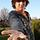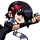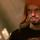## General Question# Integral [x^3 + 1]^2 dx = ? I found that it was [(x^3 + 1)^3] / 9x^2, but that is wrong? Details inside.

Asked by HeroicZach (195) May 3rd, 2009

So I solved this question by going through these steps:

First, I used the power rule of integration. So, it then looked like this:

[(x^3 + 1)^3] / 3

So then I realized that when you derive that, the chain rule applies to the portion in the numerator. To compensate, I added the value of the derivative of the part in parenthesis to which the chain rule applies, 3x^2, into the denominator. I got my answer, then, which was:

[(x^3 + 1)^3] / 9x^2

This is the incorrect answer, apparently. Is there somewhere where I took a wrong turn? It must be somewhere in my recognition of the power rule. Do you have any help for me?

Thank you!

Observing members: 0Composing members: 0Expand this using FOIL. You will get three distinct terms and it will be a very easy integral. The answer should be (x^7)/7 + (x^4)/2 + x

Ivan (13479)“Great Answer” (1) Flag as…@Ivan I see how that works. But why does my method not work? That’s what’s not making sense to me. Thanks for your answer, too!

HeroicZach (195)“Great Answer” (0) Flag as…Well, if you simplify your answer, you get something that’s sort of close…

(x^7)/9 + (x^4)/3 + x/3 + 1/(9x^2)

I think you are using a rule that doesn’t really exist.

Ivan (13479)“Great Answer” (0) Flag as…You attempted to use the chain rule, by dividing by the derivative of the inside function to correct for the result of the chain rule. You can only do this if the derivative of the inside function is a constant. If the differential equation were (2x+1)^2, then you could use your logic and get (2x+1)^3/(2*3). But look at your answer: if you were to try to find its derivative, you could not just use the chain rule with (x^3+1) as the inside function; you would have to use the quotient rule, because 9x^2 is a function of x as well. You can never divide by a non-constant term to correct for another. To get the actual answer, expand as Ivan says.

Jayne (6756)“Great Answer” (2) Flag as…or